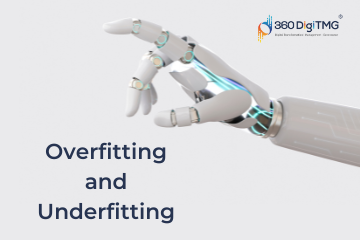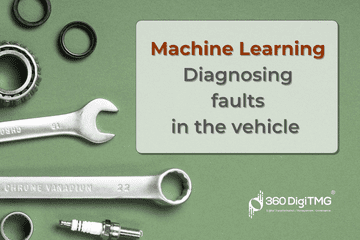Home / Blog / Machine Learning / K means clustering

# K means clustering

• May 09, 2022
• 5923
• 49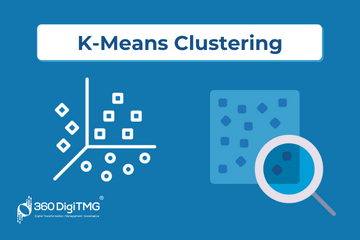### Meet the Author : Mr. Bharani Kumar

Bharani Kumar Depuru is a well known IT personality from Hyderabad. He is the Founder and Director of Innodatatics Pvt Ltd and 360DigiTMG. Bharani Kumar is an IIT and ISB alumni with more than 17 years of experience, he held prominent positions in the IT elites like HSBC, ITC Infotech, Infosys, and Deloitte. He is a prevalent IT consultant specializing in Industrial Revolution 4.0 implementation, Data Analytics practice setup, Artificial Intelligence, Big Data Analytics, Industrial IoT, Business Intelligence and Business Management. Bharani Kumar is also the chief trainer at 360DigiTMG with more than Ten years of experience and has been making the IT transition journey easy for his students. 360DigiTMG is at the forefront of delivering quality education, thereby bridging the gap between academia and industry.

Unsupervised learning and supervised learning are the two main categories of machine learning.

Clustering in this context comes under unsupervised learning, as there are no established prediction classes. It is a small group that is also known as segmentation.

In exploratory data mining, it performs the primary duty.

### Types of clustering

• Partition based clustering

Evaluate based on certain criteria Examples : K means, K- medoids, Clarans

Hierarchical approach

Creating a hierarchical decomposition of a set of data Examples: Birch, Agnes, Diana

• Density-based clustering

Based on connectivity and density functions, an algorithm is built. Examples: DBSCAN

• Grid-based algorithm

Based on a multilevel granularity structure, an algorithm is built.

### K means algorithm :

K denotes that the method calculates centroids and keeps going until it finds the ideal centroid. Another name for it is a flat clustering algorithm. The number of clusters is indicated by the letter K in K means.

We must learn from the facts. Based on distance, it employs a non-hierarchical method to identify spherical clusters that are mutually exclusive. The procedure for dividing a data set into k unique, nonoverlapping clusters is straightforward and elegant. This approach involves grouping data points into clusters in order to minimise the sum of the squared distances between each data point and the centroid.

### Working of k means algorithm

• Choose a K value that has to be generated by the algorithm
• Randomly assign a number between 1 and k to each observation, and categorize the data based on the number of data points.
• These serve as initial cluster assignments(cluster centroids are calculated)
• For each record, assign it to the cluster with the closest centroid
• Re-calculate centroids for the “losing” and“receiving” clusters.
• Repeat 2 and 3 steps until no more reassignment is necessary.

It halts creating or retrieving clusters when either:

Since the clustering was successful, the centroids of newly created clusters are stable; their values do not change any more.

The Expectation-Maximization approach is used by K-means to overcome the issue. Data points are assigned to the closest cluster using the expectation step, and the centroid of each cluster is determined using the maximisation step.

### Initial partitions can be obtained by either

• user-specified initial partitions.
• user-specified initial centroids, or< /li>
• random partitions.

### Implementation of k means in graphical form

Step1: Let us pick k clusters, i.e., K=4, to separate the dataset and assign it to clusters. We will select 4 random places to function as the cluster’s centroid.

Step 2:Now, each data point will be assigned to a scatter plot depending on its distance from the nearest K-point or centroid.

Step 3: All the data points shuffle and jump from one cluster to another concerning the nearest centroid. For example, a + symbol becomes a triangle, or a triangle becomes a circle … This process is iterated till all the data points are fixed in clusters whose centroids are closer.

Step4: This iteration process will stop when all centroids are static and no further changes are required.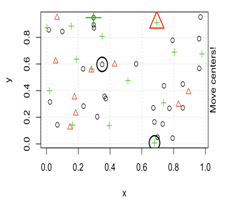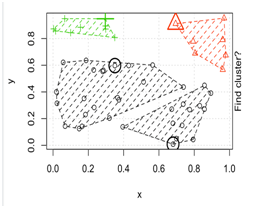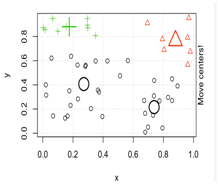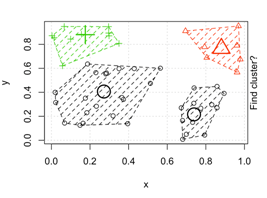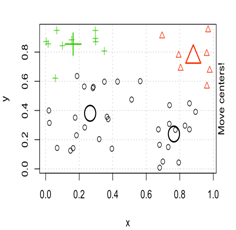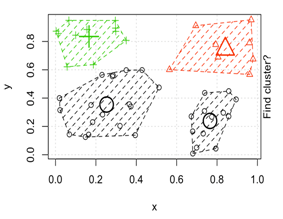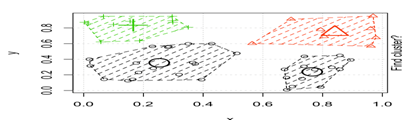### Implementing in python :

This is an illustration of 100 random integers from 0 to 1.

In this example, we'll build a scatter plot first, then use the k-means algorithm to see the outcomes.

We will bring in necessary items.

import pandas as pd

import matplotlib.pyplot as plt

from sklearn.cluster import KMeans

from scipy.spatial.distance import cdist

import numpy as np

# Generating random uniform numbers

X = np.random.uniform(0,1,1000)

Y = np.random.uniform(0,1,1000)

# converting the data to data frame

df_xy =pd.DataFrame(columns=["X","Y"])

df_xy.X = X

df_xy.Y = Y

Visualising the data

df_xy.plot(x="X",y = "Y",kind="scatter")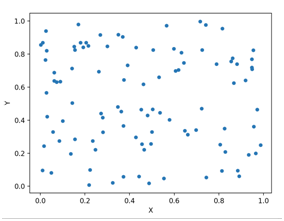To create a K – means object while specifying the number of clusters

model1 = KMeans(n_clusters=5).fit(df_xy)

df_xy.plot(x="X",y = "Y",c=model1.labels_,kind="scatter",s=10,cmap=plt.cm.coolwarm)

centres = model1.cluster_centers_

plt.scatter(centres[:, 0], centres[:, 1], c='blue', s=100, alpha=0.9);

plt.show()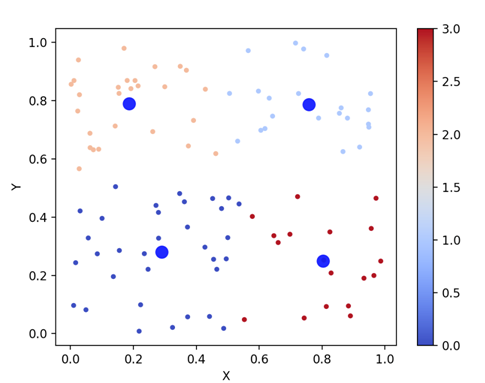### Selection of K

* Using Scree plot / elbow curve

making a list of all possible K values inside the sum of squares and choosing the K value with the steepest bend—almost an elbow—in the curve. This provides an indication of the optimal number of clusters for the dataset.

Sqrt(n/2) may also be used to do this, where n is the number of clusters, although it would not be as successful for really big datasets.

To identify which cluster assignment is preferable, we may utilise MSE.

TWSS = [ ]

k = list(range(2, 9))

for i in k:

kmeans = KMeans(n_clusters = i)

kmeans.fit(df_xy)

TWSS.append(kmeans.inertia_)

TWSS

# Scree plot

plt.plot(k, TWSS, 'ro-');plt.xlabel("No_of_Clusters");plt.ylabel("total_within_SS")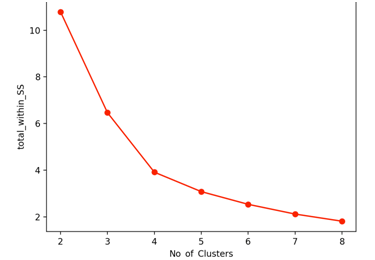From the above plot we can try with 4 clusters.

### Calculation of within and Between

p class="para_blog">within's: similarity between records within the cluster

"less the distance more the similarity".

Betweenness: Dissimilarity between the records of different clusters

"more the distance = less the similarity =better the grouping".

Silhouette Coefficient: It is a score that helps in evaluating the goodness of cluster technique whose values are in the range of -1 to +1

1: represents clusters apart from each other.

0: represents clusters are indifferent.

-1: represents clusters that are allocated in the wrong way.

• Easy to implement
• Fast( for small k)
• Clusters can be recalibrated.
• Computationally fast for large data sets.

• Initial cluster choices and order of data strongly affect results.
• Requires known 'k' clusters ; can be difficult to choose
• Can be difficult to reproduce results due to random initial centroid choice
• Applicable only when mean is defined.
• It is sensitive to rescaling

### Conclusion :

The best clustering method is K means, however it produces different results each time the algorithm is performed, earning it the label NONDETERMINISTIC ALGORITHM.K-means clustering is an unsupervised algorithm that uses the input data as-is and does not require a tagged response. The best course of action when clusters have a spherical structure is to select K means.

Click here to learn Data Science Course, Data Science Course in Hyderabad, Data Science Course in Bangalore

### Data Science Training Institutes in Other Locations